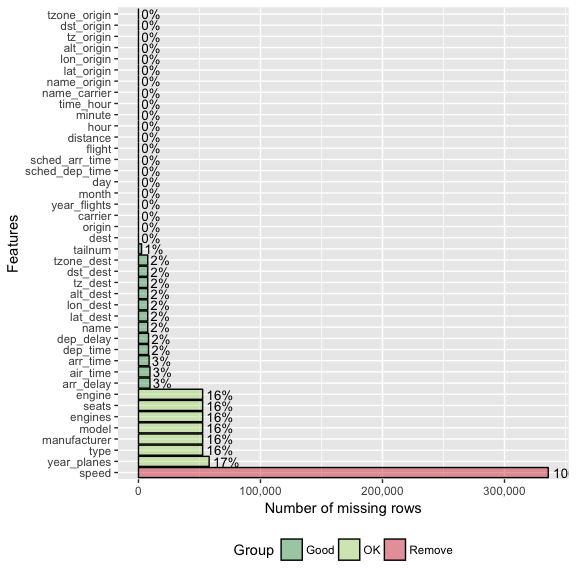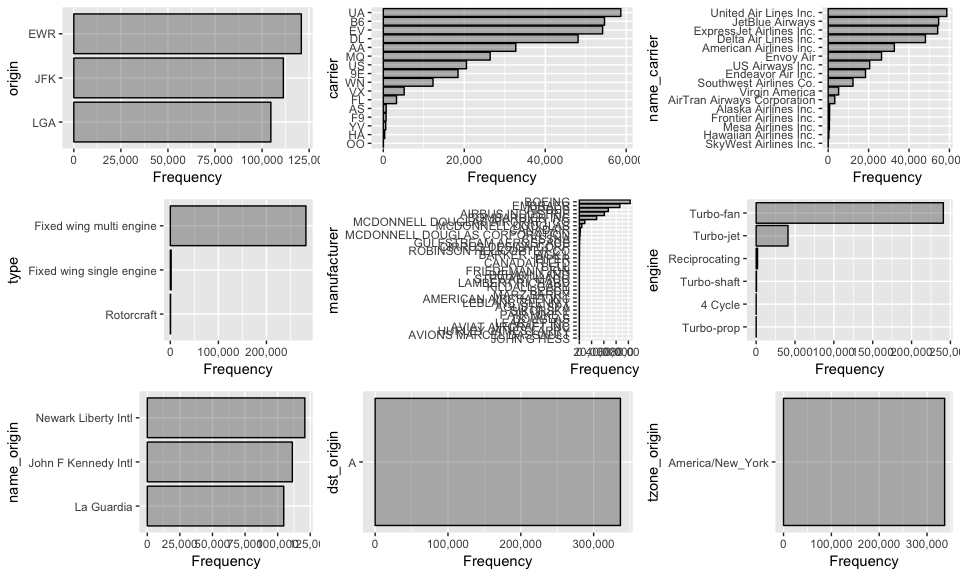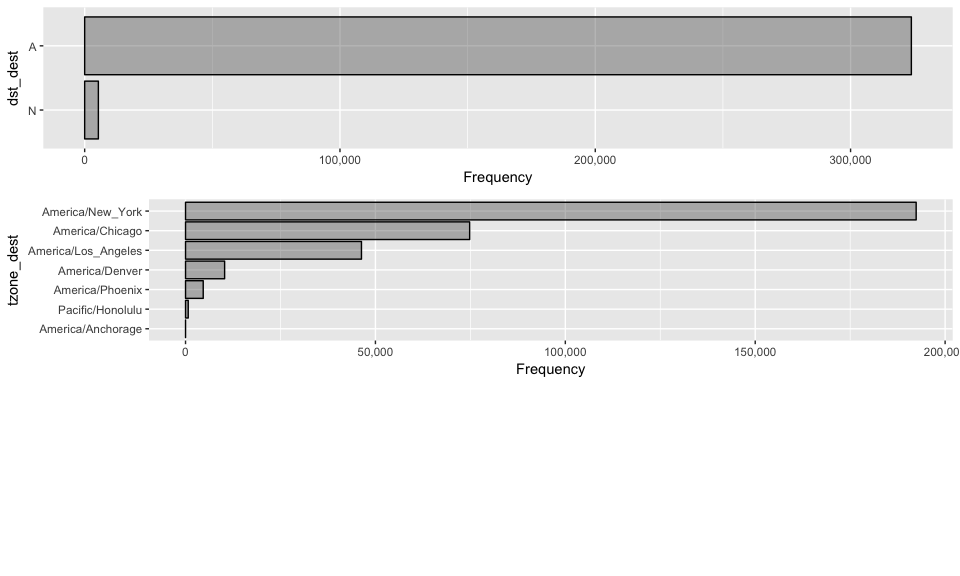# Introduction to DataExplorer

#### 2018-01-09

There are 3 main goals for DataExplorer:

1. Exploratory Data Analysis (EDA)
2. Feature Engineering
3. Data Reporting

The remaining of this guide will be organized in accordance with the goals. As the package evolves, more content will be added.

## Data

We will be using the nycflights13 datasets for this document. If you have not installed the package, please do the following:

install.packages(“nycflights13”) library(nycflights13)

There are 5 datasets in this package:

• airlines
• airports
• flights
• planes
• weather

If you want to quickly visualize the structure of all, you may do the following:

``````library(DataExplorer)
data_list <- list(airlines, airports, flights, planes, weather)
plot_str(data_list)``````

You may also try `plot_str(data_list, type = "r")` for a radial network.

Now let’s merge all tables together for a more robust dataset for later sections.

``````merge_airlines <- merge(flights, airlines, by = "carrier", all.x = TRUE)
merge_planes <- merge(merge_airlines, planes, by = "tailnum", all.x = TRUE, suffixes = c("_flights", "_planes"))
merge_airports_origin <- merge(merge_planes, airports, by.x = "origin", by.y = "faa", all.x = TRUE, suffixes = c("_carrier", "_origin"))
final_data <- merge(merge_airports_origin, airports, by.x = "dest", by.y = "faa", all.x = TRUE, suffixes = c("_origin", "_dest"))``````

## Exploratory Data Analysis

Exploratory data analysis is the process to get to know your data, so that you can generate and test your hypothesis. Visualization techniques are usually applied.

You can easily check the basic statistics with base R, e.g.,

``````dim(final_data)
summary(final_data)
object.size(final_data)``````

### Missing values

Real-world data is messy. After running the basic descriptive statistics, you might be interested in the missing data profile. You can simple use `plot_missing` function for this.

``plot_missing(final_data)``You may also store the missing data profile with `missing_data <- plot_missing(final_data)` for additional analysis.

### Distributions

To visualize distributions for all discrete features:

``plot_bar(final_data)``
``````## 5 columns ignored with more than 50 categories.
## dest: 105 categories
## tailnum: 4044 categories
## time_hour: 6936 categories
## model: 128 categories
## name: 102 categories``````To visualize distributions for all continuous features:

``plot_histogram(final_data)``